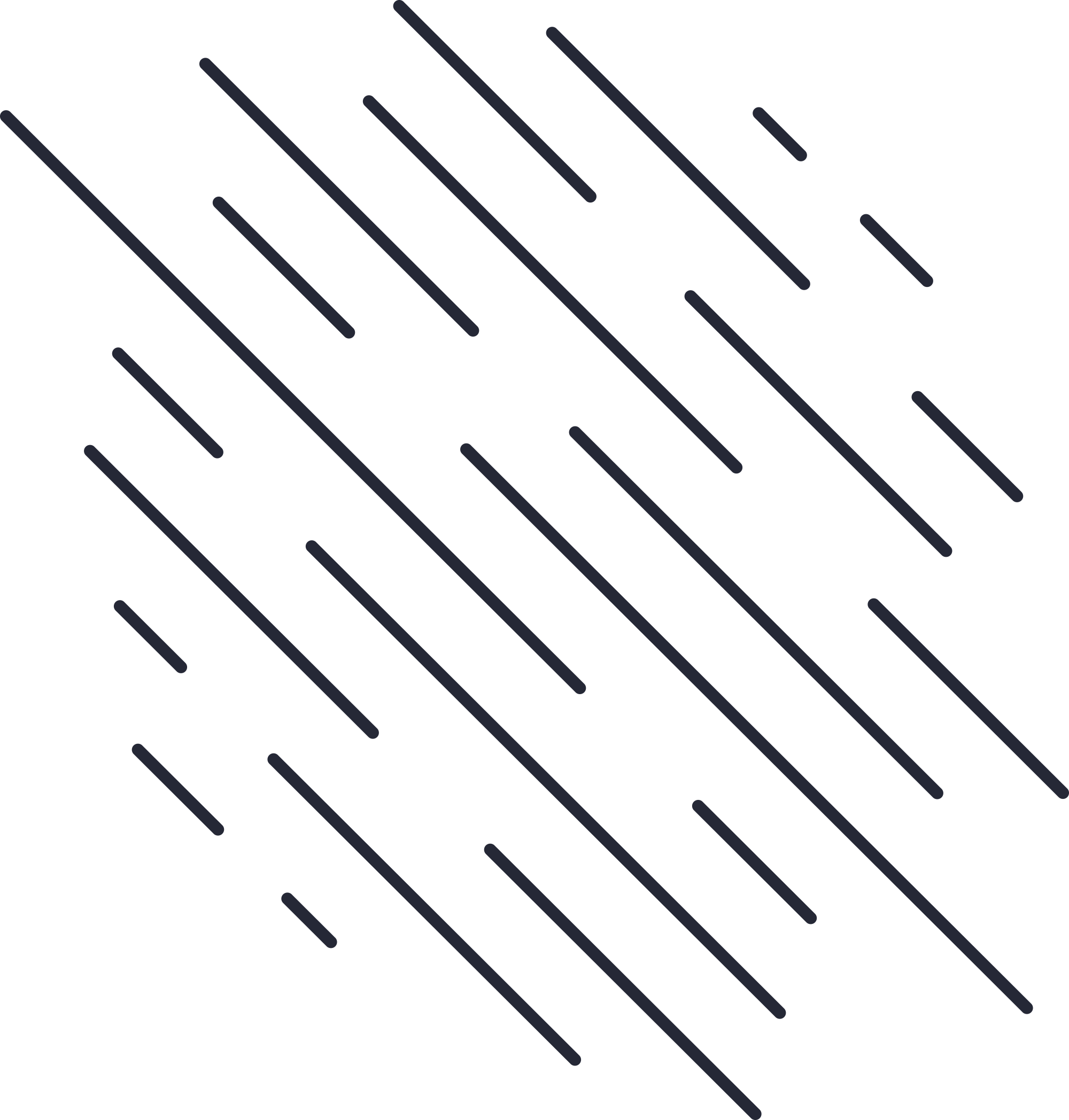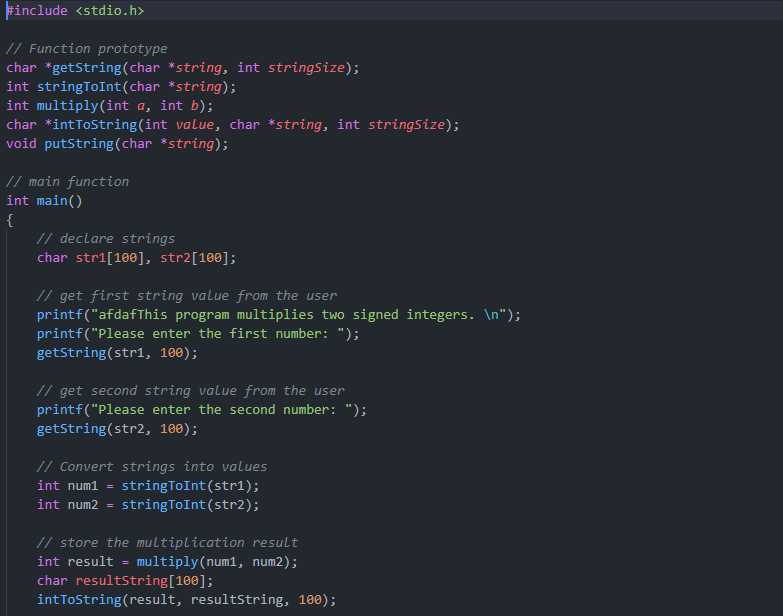# Final Program in hll

Programming CWrite a program in the C Programming Language to perform the following actions.  Write separate procedures or functions for each of these
operations:

(1) input a string using character input operations

(2) convert a string to a signed integer numeric value in two's-complement representation representing the same value in decimal and checking that all characters in the string are digits with a possible preceding negative-sign (which is the same character as a dash or hyphen) -- this function should indicate if the input string cannot be successfully be represented as a signed integer numeric value,

(3) take two signed integer numeric values in two's-complement representation (these are named the multiplicand and the multiplier) and multiply them to produce a signed integer numeric product in two's-complement representation (at your option, the product may be either at the same precision as the multiplicand and the multiplier or may be in twice the precision of the multiplicand and the multiplier) -- it is not necessary to detect overflow,

(4) convert a signed integer numeric value in two's-complement representation to a string representing the same value in decimal (note that this value may be negative), and

(5) output a string using character output operations. These functions are specified to perform the same way that the functions required for the final project perform.

The minimum number you need to be able to read is -32768 and the maximum number you need to be able to read is 32767.  You're welcome to accept numbers that span a larger range.  The minimum precision for all signed integer numeric values must be able to represent values from -32768 to 32767, inclusively.  Note that integer types in C on all modern computers use two's-complement representation; therefore, you are welcome to use "int" as the data type for your numbers. Please do *not* create arrays of bits to represent numeric values.

All input characters are encoded using the ASCII character-encoding scheme.

None of these functions should use any library methods to perform their computation.  That is, do not use built-in calls to perform the string-to-value conversions (or vice versa); this conversion should be written from scratch.  You are allowed to use library functions for input/output operations for characters, but not for either strings or for integers (i.e., fputc(c, stream), putc(c, stream), and putchar(c) are allowed for output, but fputs(s, stream), puts(s), printf("%s", s), and printf("%d", i) are *not* allowed; fgetc(stream), getc(stream), and getchar() are allowed for input, but fgets(s, n, stream), gets(s), scanf("%s", s), and scanf("%d", &i) are *not* allowed).  Also, do not use the multiply operator to perform multiplication. Your algorithm for multiplication should use an efficient shifting approach.  None of your code should use a multiply, divide, remainder, or modulo operator provided by the programming language.

A main program should exist to call these functions so that:

(1) a prompt is output asking the user to enter a value and that value is input as a string,

(2) a second prompt is output asking the user to enter a second value and that value is input as a string,

(3) the input strings are converted to integer numeric values and any errors are output to the user if the strings do not contain strictly decimal digits,

(4) the two input values are multiplied by each other to produce a product,

(5) the product is converted into a string representing that decimal value,

(6) the product string is output preceded by an appropriate string describing the output.

.

Your interaction with the main program might look like:

This program multiplies two signed integers.  Please enter the first number: 3
Please enter the second number: 4
The product of 3 and 4 is 12.
This program multiplies two signed integers.  Please enter the first number:

Function/procedure/method declarations should be:

(1) Input a string using character input operations:

(2) Convert a string to a signed integer numeric value:

int stringToInt(char *string);

/* perform an operation similar to "long strtol(const char *str,
*   char **endptr, int base)"
* sample input: "1234"
* sample output: 1234
*
* Parameters:
*   string pointer to a nul-terminated char array in memory
*   the initial characters of which are to be converted
* into a signed int
*
* Return value:
* signed int value of the initial characters in the
* "string" nul-terminated string
*/

(3) Multiply two signed integer numeric values:

int multiply(int a, int b);

/* signed multiplication; don't use the multiplication operator
*
* Parameters:
*   a signed int representing the multiplicand
*   b signed int representing the multiplier
*
* Return value:
* signed int value of the multiplication product
*/

(4) Convert a signed integer numeric value in two's-complement
representation to a string representing the same value in decimal:

char *intToString(int value, char *string, int stringSize);

/* perform an operation similar to "int sprintf(char *s, const char *format,
*   ...)" with a format string of "%d"
* sample input: 1234
* sample output: ['1,'2','3','4','\n']
*
* Parameters:
*   value signed int value to be converted into an ASCII
*   string
*   string pointer to a char array in memory in which ASCII
*   characters representing the "value" are returned as
* a nul-terminated string
*   stringSize size of the "string" char array in memory
*
* Return value:
* identically the same pointer to the char array in
* memory in which the ASCII string is returned that
* was passed in as the first parameter
*/

(5) Output a string using character output operations:

void putString(char *string);

/* output a string to stdout.  Perform an operation similar to
*   "int fputs(const char *s, FILE *stream)"
* should call "int fputc(int c, FILE *stream)" to perform the output
*
* Parameters:
*   string pointer to a nul-terminated char array in memory
*   the characters of which are to be output
*
* Return value:
* none
*/

### Lets Work Together